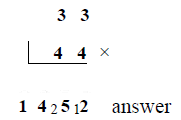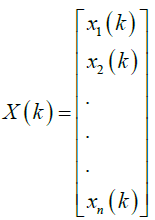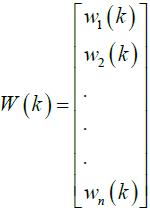Implementation of LMS-ALE Filter Using Vedic Algorithm | OMICS International
Journal of Electrical & Electronic Systems

# Implementation of LMS-ALE Filter Using Vedic Algorithm

Joseph Jintu K1* and Purushotham U2

1VLSI & Embedded systems PESIT Bangalore, India.

2Department of Electronics & Communications PESIT Bangalore, India.

*Corresponding Author:
Joseph Jintu K
VLSI & Embedded systems
PESIT Bangalore, India
Tel: 080661 86610
E-mail: [email protected]

Received Date: July 20, 2015; Accepted Date: August 19, 2016; Published Date: August 26, 2016

Citation: Joseph Jintu K, Purushotham U (2016) Implementation of LMS-ALE Filter Using Vedic Algorithm. J Electr Electron Syst 5:192. doi: 10.4172/2332-0796.1000192

Copyright: © 2016 Joseph Jintu K, et al. This is an open-access article distributed under the terms of the Creative Commons Attribution License, which permits unrestricted use, distribution, and reproduction in any medium, provided the original author and source are credited.

Visit for more related articles at Journal of Electrical & Electronic Systems

#### Abstract

ALE or adaptive line Enhancers are special kinds of adaptive filters widely used in noise cancellation circuits. In circuits where we don’t have any prior knowledge of signal and noise, fixed filters unit never works good. Among adaptive filter ring algorithms LMS algorithm is very common, in our work also we use LMS algorithm. LMS-ALE filters removes the sinusoidal noise signals present in the channel by calculating the filter coefficients in every iteration. LMSALE filter has large number of multiplier units. FFT or Fast Fourier Transform blocks present in LMS algorithm again consist of large array of multiplier units. Optimization of LMS-ALE filter lies must start from optimization of multiplier blocks. Here we use Vedic “Vertical and crosswise algorithm” for multiplier design. When compared to conventional booth multiplier based LMS-ALE filter units, Vedic multipliers gives more performance in areas like resource utilization, power requirement, delay etc. The work includes designing Vedic multipliers, complex Vedic multipliers, redesigning Radix-8 FFT using Vedic multipliers, redesigning LMS block using Vedic FFT, redesigning LMS ALE filter using Vedic multipliers and Vedic LMS blocks. Major part of design is done in verilog using Xilinx ISE design suite. ADC block present in LMS-ALE filter is done in Matlab version 2013.

#### Abstract

ALE or adaptive line Enhancers are special kinds of adaptive filters widely used in noise cancellation circuits. In circuits where we don’t have any prior knowledge of signal and noise, fixed filters unit never works good. Among adaptive filter ring algorithms LMS algorithm is very common, in our work also we use LMS algorithm. LMS-ALE filters removes the sinusoidal noise signals present in the channel by calculating the filter coefficients in every iteration. LMSALE filter has large number of multiplier units. FFT or Fast Fourier Transform blocks present in LMS algorithm again consist of large array of multiplier units. Optimization of LMS-ALE filter lies must start from optimization of multiplier blocks. Here we use Vedic “Vertical and crosswise algorithm” for multiplier design. When compared to conventional booth multiplier based LMS-ALE filter units, Vedic multipliers gives more performance in areas like resource utilization, power requirement, delay etc. The work includes designing Vedic multipliers, complex Vedic multipliers, redesigning Radix-8 FFT using Vedic multipliers, redesigning LMS block using Vedic FFT, redesigning LMS ALE filter using Vedic multipliers and Vedic LMS blocks. Major part of design is done in verilog using Xilinx ISE design suite. ADC block present in LMS-ALE filter is done in Matlab version 2013.

#### Keywords

ALE filter; LMS algorithm; Vedic algorithm; Fast multipliers; Booth multipliers; Radix 8 FFT; Verilog; Xilinx ISE Design suite; Matlab

#### Introduction

In physical environment noise is automatic signal, in all kinds of signal generated there is some kind of unwanted noise signals, when such a signal is amplified in a communication channel both noise and desired signal gets amplified equally, it reduces the clarity of communication system, hence noise cancellation is inevitable. ALE Filter is a most common noise cancellation system. It uses some kind of adaptive algorithm [LMS algorithm]. LMS adaptive block consist of FFT and inverse FFT blocks as it handles the signal in frequency domain. Multipliers are the most repeated block in LMS-ALE filter, to optimize the performance we need to do the optimization from basic multiplier block. In this work we use Vedic algorithm for doing the multiplication. Compared to conventional booth algorithm Vedic multipliers requires less partial product adders, hence it improves the performance in terms of delay, resource utilization and power requirement [1-5].

#### Methodology

ALE filter

Adaptive Line Enhancer [ALE] filter optimized to remove sinusoidal noise signals present in the channel. In Figure 1, s(n) represents the desired signal, n(n) denotes the noise signal, z- represents de-correlation function, and the block is followed by an adaptive predictor unit block. De-correlation eliminates any kind of correlation that may exist between the noise samples. Predictor can make prediction on the sinusoidal component of the noise signals and system will adaptively minimize the instantaneous squared error output. Inputs to the adaptive filter or predictor unit is units behind the original input signal. Therefore, in order to time align the enhanced signal, Å�(n) with the input signal, x(n) the adaptive filter must be able to ‘predict ahead’ in time by optimizing its filter coefficients in a least squares sense, hence the instantaneous squared error is minimized .

Vedic maths

Vedic mathematics or else called Indian mathematic is originated in Indian sub-continent at 1200BC. Ancient time significant growth to this field is done by great scholars like Aryabhata, rahmagupta, Mahavira, Bhaskara II, Nilakantha Somayaji etc. the decimal number system that we are using today is first record in Indian mathematic books. Vedic maths are the list of mental mathematical calculation techniques described in Vedas. Those mental techniques are combined together and described in special text called “vedic mathematics” .

Vedic multiplication algorithm

Vertical and crosswise algorithm is one among the 16 Vedic sutras mentioned in the Vedic mathematics. Vertical and crosswise algorithm is also called as Urdhva Tiryagbhyam. I use this sutra to optimize the multiplier performance. Multiplication example using vertical and crosswise algorithm is given below. We want to multiply 33 by 44:Multiplying vertically on the right we get 3 × 4=12, so we put down 2 and carry 1 (written 12 above). Then we multiply crosswise and add the two results: 3 × 4 + 3 × 4=24. Adding the carried 1 gives 25 so we put 5 and carry 2 (25). Finally we multiply vertically on the left, get 3 × 4=12 and add the carried 2 to get 14 which we put down .

Complex vedic multiplication

The flow chart in Figure 2 shows the procedure to compute the addition of any two signed numbers, Where x1 denotes both the input say a, b have same sign, if both have same sign then x1 take 1, Else x1=0. When x1 is high it looks like simple unsigned number addition. The result takes the sign of the inputs. If x1=0, we need to compute y1 value. Y1 is equal to 1 if first input says 1 > second input b, else it takes 0. If y1=1, then I perform a-b operation as a is larger than b, else I perform b-a operation, which implies b is larger than a. The output takes the same sign as a larger input operand.

The flow chart in Figure 3 shows the procedure to compute the subtraction of any two signed numbers, Where x1 denotes both the input say a, b have same sign, if both have same sign then x1 take 1, Else x1=0. When x1 is high, I need to compute y1 value. Y1 is equal to 1 if first input says 1 > second input b, else it takes 0. If y1=1, then I perform a-b operation as a is larger than b, else I perform b-a operation, which implies b is larger than a. The output takes the same sign as a larger input operand. If x1=0, it looks like simple unsigned number addition. The result takes the sign of the inputs .

Vedic 4 × 4 complex multiplier

Figure 4 shows the 4 × 4 bit multiplication using Vedic algorithm . It consists of four 2 × 2 Vedic multiplier units, two 4-bit [N bit adder] adders and one 2-bit [N/2 bit adder] units. The orange colored circles indicate the selected operand for multiplication. q [1:0] is equal to Q [1:0], the final result .

LMS algorithm

LMS algorithm is the basic adaptive algorithm available, this algorithm helps any system to mimic a desired filter by generating weights adaptively according to the value to error signal. Figure 5

The LMS algorithm is very useful and easy to compute [8,9]. The LMS algorithm will perform Ill, if the adaptive system is an adaptive linear combiner, as Ill as, if both the n-dimensional input vector X(k) and the desire output

d(k) are available in each iteration, where X(k) isAnd the n-dimensional corresponding set of adjustable weights W(k) isBy having the input vector X(k), the estimated output y(k), can be computed as a linear combination of the input vector X(k) with the weight vector W(k) as

y(k) = XT (k)W(k)

Thus, the estimated error e(k), the difference between the estimated output y(k), and the desired signal d(k), can be computed as

e(k) = d(k) - y(k) = d(k) - XT (k)W(k)

#### Results

The above figure is the simulation result obtained when 2 complex numbers each of 6 bit wide are multiplied, a and b are the input, each has two components a_r, a_i and b_i, b_r. B_r, a_r represents the real part and a_i, b_i represents the imaginary part. A_r_s, a_i_s and b_i_s, b_r_s represents the sign of real part and imaginary part of inputs respectively. The output obtained is c, it also has two components c_r [real part] and c_i [imaginary part].

For smaller modules booth algorithm based multipliers consumes larges resources, for 4 bit multiplier it uses 73 and 19, 4 input LUTs when implemented Booth algorithm and Vedic algorithm. 19 and 42 numbers of occupied slices when implemented using Vedic algorithm and Booth algorithm. When the input gets wider or module becomes larger Vedic algorithm based design consumes larges resources and booth algorithm based design consumes lesser resources.

Figure 6 shows the power utilization report for Radix 8 FFT  implemented using Vedic algorithm and Booth algorithm. These results are obtained from the X-power analyzer tool of Xilinx ISE software. For radix 8 FFT designed using Vedic algorithm the total power utilization is 194mw, it consist of three 16 bit Vedic multiplier and two 8 bit multiplier. Hence the total power utilization is sum of power utilization of each multiplier units. For Radix 8 FFT implemented using Booth algorithm the total power utilization is around 211mw (Figures 7 and 8).

Figure 6: Power utilization comparison Graph of Radix 8 FFT implemented through Vedic algorithm and Booth algorithm.

#### Conclusion

The Fast multiplier design using Vedic algorithm has outstanding performance features in resources utilization, power requirement, delay taken, and area requirement. In this work a generic N × N bit Vedic multiplier which can perform both signed and unsigned multiplication are designed in Xilinx using verilog. An FFT module which can perform N × N bit Fourier transformation is designed in Xilinx using the Vedic multipliers designed earlier. An ADC module which takes audio input from system, converts the floating point value to 18 bit binary value are modeled in Matlab. This binary value becomes the input for the ALE. ALE block is designed in Xilinx using verilog language. The multiplier units in Ale are redesigned using Vedic multipliers. The comparison results between Vedic implementations and conventional implementation are also generated for each stage.

#### References

Select your language of interest to view the total content in your interested language

### Article Usage

• Total views: 9586
• [From(publication date):
September-2016 - Jan 18, 2020]
• Breakdown by view type
• HTML page views : 9461Can't read the image? click here to refresh